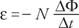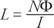## Electromagnetic Induction

The finding that electric current can produce magnetic fields led to the idea that magnetic fields could produce electric currents. The production of emfs and currents by the changing magnetic field through a conducting loop is called induction.

## Magnetic flux

The magnetic field through a loop can be changed either by changing the magnitude of the field or by changing the area of the loop. To be able to quantitatively describe these changes, magnetic flux is defined as Φ = BAcosθ, where θ is the angle between B and the direction perpendicular to the plane of the loop (along the axis of the loop).

Changing the magnetic flux through a loop of wire induces a current. Faraday's law states that the emf induced in a wire is proportional to the rate of the flux through the loop. Mathematically,where N is the number of loops, ΔΦ is the change of flux in time, Δ t. The minus sign indicates the polarity of the induced emf.

The preceding equation is easy to use when the flux is set up by an electromagnet. If the electromagnet is turned on or off, the induced emf is equal to the number of turns in the loop times the rate of change of flux. The flux might also be changed through a loop altering the size of the loop. Imagine a slide wire, as shown in Figure 1, where l is the length of the wire that moves in contact with the U‐shaped wire. In this case, ε= Blv, where v is the velocity of the sliding length.

Note that this induced emf is indistinguishable from that of a battery and that the current is still just the rate of the motion of charges; therefore, Ohm's law and other relationships for currents in wires are still valid.

## Lenz's law

The direction of the induced current can be found from Lenz's law, which states that the magnetic field generated by the induced emf produces a current whose magnetic field opposes the original change in flux through the wire loop. Again, consider Figure and assume the slide is moving to the right. The x shapes indicate that B is into the page; thus, when the slide moves to the right, the field through the slide will get greater into the page. (The change in flux is the crucial quantity.) The magnetic field from the induced current will be directed out of the page because it will oppose the change in flux. Use the second‐hand rule and place the curl of the fingers out of the page at the center of the loop. The direction of the thumb indicates that the current will flow counterclockwise. (It is not correct to state that the current is to the right because it is to the left on the top of the loop.) Conversely, if the slide moves to the left, B will decrease through the loop. The change in flux will be out of the page, and the induced current will be clockwise. The same analysis is used if an electromagnet is turned on or off.Figure 1 A slide wire circuit generates emf.

Lenz's law is also a conservation law. If the magnetic field generated by the induced current could be in the same direction as the original change in flux, the change would get larger and the induced current greater. This impossible condition would be a better energy exchange than a perpetual motion machine.

## Generators and motors

Generators and motors are applications of electromagnetic induction. Figure illustrates a simple electric generator.Figure 2 A simple electric generator.

The crank represents a mechanical method of turning the loop of wire in a magnetic field. The change in magnetic flux through the loop generates an induced current; thus, the generator converts mechanical energy into electrical energy. The operation of a motor is similar to that of a generator but in reverse. The motor has similar physical components except that the electric current supplied to the loop exerts a torque, which turns the loop. The motor, therefore, converts electrical energy into mechanical energy.

## Mutual inductance and self-inductance

Mutual inductance occurs when two circuits are arranged so that the change in current in one causes an emf to be induced in the other.

Imagine a simple circuit of a switch, a coil, and a battery. When the switch is closed, the current through the coil sets up a magnetic field. As the current is increasing, the magnetic flux through the coil is also changing. This changing magnetic flux generates an emf opposing that of the battery. This effect occurs only while the current is either increasing to its steady state value immediately after the switch is closed or decreasing to zero when the switch is opened. This effect is called self‐inductance. The proportional constant between the self‐induced emf and the time rate of change of the current is called inductance (L) and is given byThe SI unit for inductance is the henry, and 1 henry = 1(Vs/A).

Using Faraday's law, inductance can be expressed in terms of the change of flux and current:where N is the number of turns of the coil.

## Maxwell's equations and electromagnetic waves

Maxwell's equations summarize electromagnetic effects in four equations. The equations are too complex for this text, but the concepts embodied in them are important to consider. Maxwell explained that electric and magnetic waves can be generated by oscillating electric charges. These electromagnetic waves may be depicted as crossed electric and magnetic fields propagating through space perpendicular to the direction of motion and to each other, as illustrated in Figure 3.Figure 3 An electromagnetic wave consists of perpendicular oscillating magnetic and electric fields.